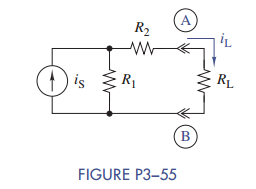### Create an Account

Home / Questions / Find the Thévenin or Norton equivalent circuit seen by RL in Figure P3−55 b Use the equ...

# Find the Thévenin or Norton equivalent circuit seen by RL in Figure P3−55 b Use the equivalent circuit found in part a to find iL in terms of iS R1 R2 and RL c Check your answer to

(a) Find the Thévenin or Norton equivalent circuit seen by RL in Figure P3−55.

(b) Use the equivalent circuit found in part (a) to find iL in terms of iS, R1, R2, and RL.Subscribe To Get Solution